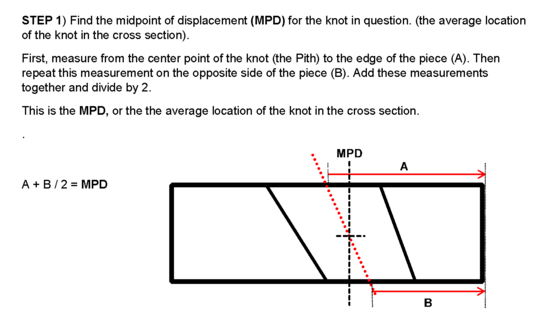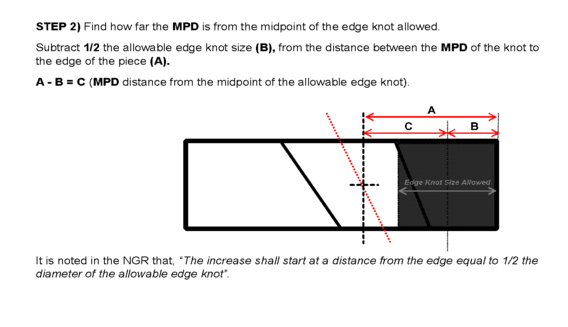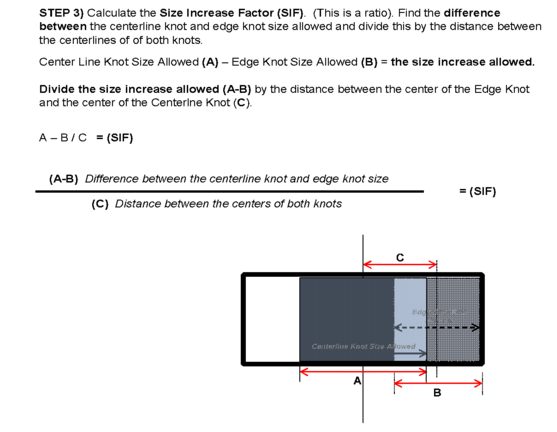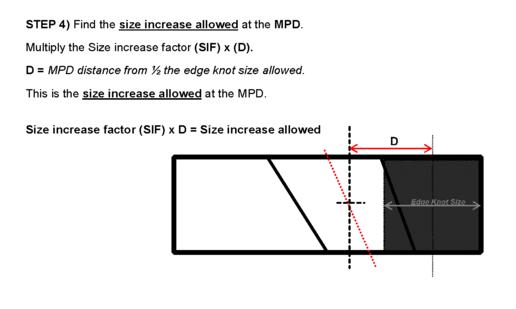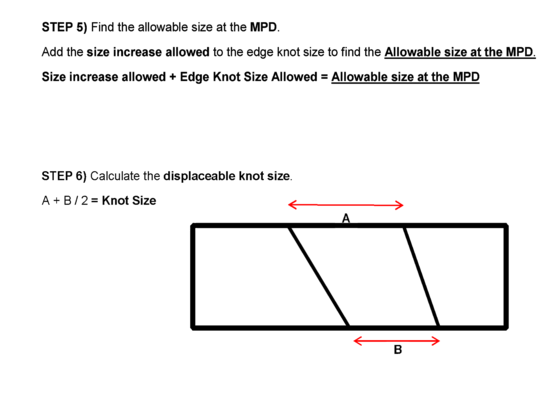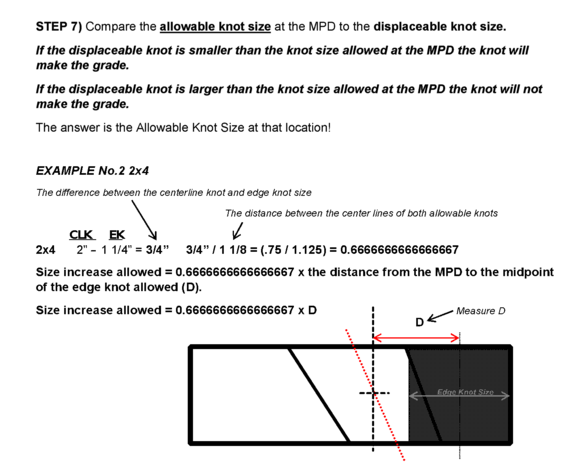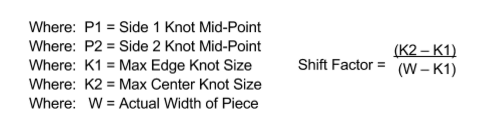Back# How to Grade Displaceable Knots

## Grading Knots Away From the Edge

Learning to grade edge knots and combination knots in dimension lumber takes a fair amount of study and practice. These type knots most often limit the grade of a piece. Once the grader has a complete understanding of how these knots are measured and limited it becomes a matter of closely judging the size of what you see and comparing this to a known limit for a known knot size. This makes it relatively easy to estimate the size, and grade accurately on edge and combination knots.

One of the most difficult types of knots to visually grade is known as a “displaceable knot.” A displaceable knot is a knot appearing on both wide faces and is located away from the edge. Visually grading a displaceable knot accurately can be challenging. It is difficult to grade visually because several calculations and measurements are required to determine the allowable knot size at various distances from the edge. However, understanding and executing the steps involved to calculate the allowable knot size at a certain distance from the edge on a few knots will make it easier for you to evaluate these knots. After learning the steps described here, you can download our Displaceable Knot Guide. This guide contains a simplified formula to calculate the allowable knot size for 2” Dimension Lumber at any distance from the edge. You can use this tool to practice and check your decisions on a few displaceable knots. It will make you a better lumber grader on the chain, or when checking lumber during an inspection.

## Determining the Allowable Knot Size

How to limit knots away from the edge is described in the National Grading Rule (NGR) for Softwood Dimension Lumber Interpretations: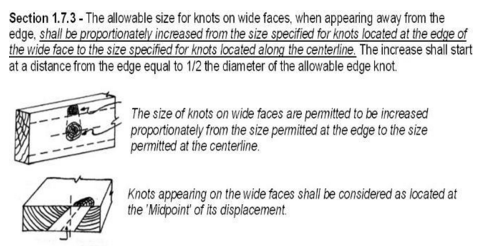The NGR Interpretation, Section 1.7.3 describes knot location and allowable knot size, but it does not detail how to calculate the allowable size at a given distance from the edge for displaceable knots.

When evaluating displaceable knots, it is important to note that the NGR states that a knot must occupy less than ½ the edge before it can be displaced, and illustrates this following in this drawing: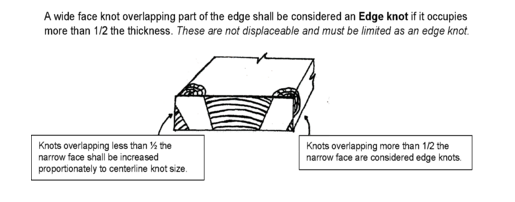## Steps for Grading Displaceable Knots

From the NGR Interpretations, we know that a knot away from the edge can be larger than the edge knot allowed, and it cannot exceed the centerline knot size allowed. The permissible increase in size (from the allowed edge knot) must be proportionate. This means the size increase allowed for the displaceable knot must be in the same proportion (ratio), as the size increase allowed over the distance, between the edge and center line knots, in each width and grade.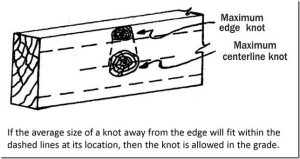To find the allowable increase, we must know how far away the displaceable knot is from the edge and then calculate how large it can be at that location. The steps necessary for finding the allowable increase in size are simply stated below.

For the size and grade in question, find the difference in the size between the edge and centerline knots allowed. Divide this difference by the distance between the centers of the allowed edge and centerline knot.

Multiply the result by the distance between the Mid-Point of Displacement of the displaceable knot and the midpoint of the allowable edge knot.

Add this result to the allowable edge knot size.

The answer is the Allowable Knot Size at that location!

## Practice, Practice, Practice

Each of these steps are explained and illustrated in detail below. You can click on each drawing to expand the illustrated explanation. A simplified formula that you can use in the field is provided in a downloadable eBook at the end. You should try these calculations on a few displaceable knots. You will be able to make much more accurate decisions with a little practice measuring and checking the grade of a few displaceable knots.